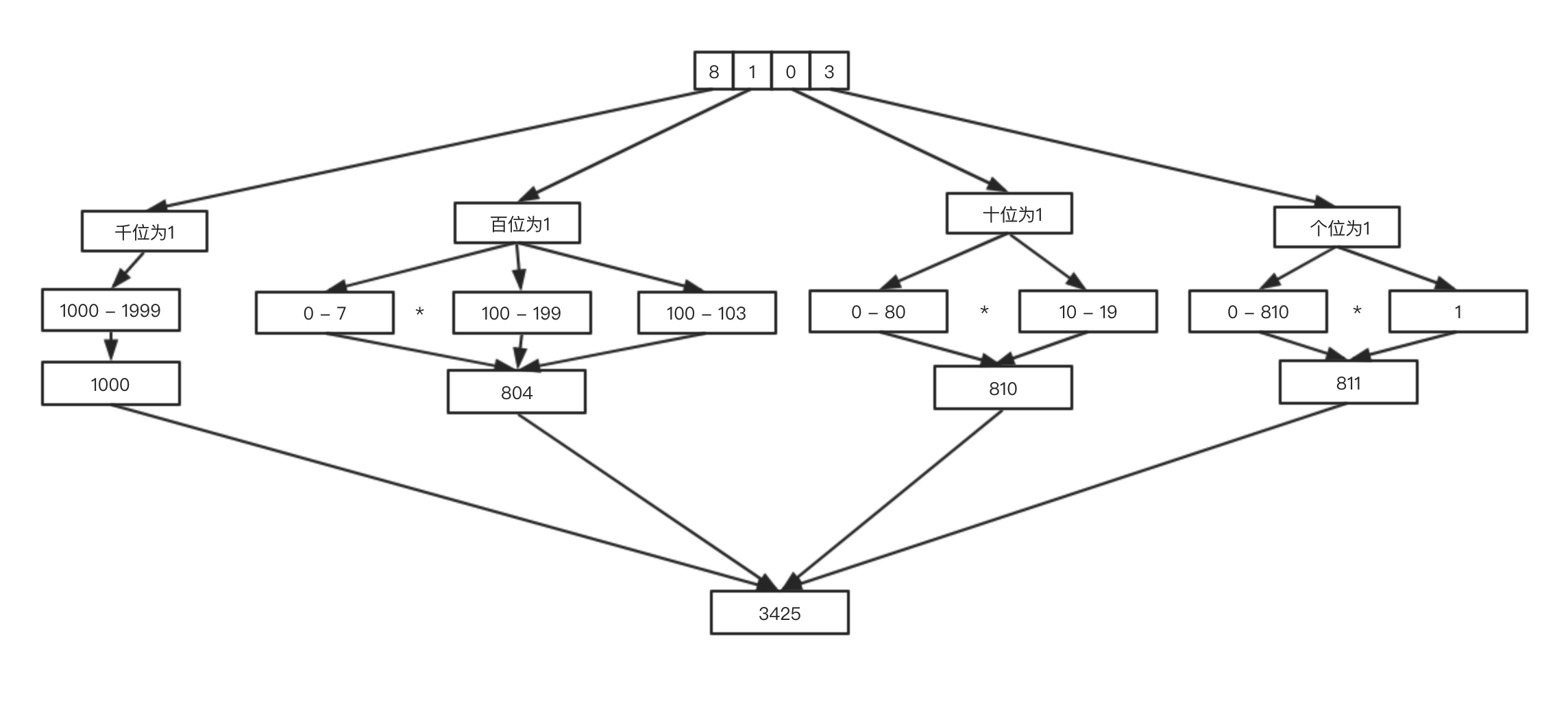## # 题目

`ACMer`希望你们帮帮他,并把问题更加普遍化,可以很快的求出任意非负整数区间中`1`出现的次数（从`1``n``1`出现的次数）。

## # 思路

• 个位 `3`: 个位已经是最低位了，所以`low`只有`1`中情况。`high`可以取`0 - 810``811`种情况，所有情况为`1 * 811 = 811`种情况。

• 十位 `0`: `low`可能为`10 - 19``10`种情况，`high`可以取`0 - 80``81`种情况，所有情况为`81 * 10 = 810`种情况。

• 百位 `1`: `low`可能为`100 - 199``100`种情况，`high`可以取`0 - 7``8`种情况;当`high``8`时，`low`还可以取`100 - 104`，所有情况为`100 * 8 + 4 = 804`种情况。

• 千位 `8`:`low`可能为`1000 - 1999``1000`种情况，当前已经是最高位了，`high`只有一种情况，所有情况为`1000 * 1 = 1000`种情况。• `c = 0` : 有 `ab*100` 种情况
• `c = 1` : 有 `ab*100 + de + 1` 种情况
• `c > 2` : 有 `(ab+1) * 100` 种情况

`c``abcde``3`位数：

• `ab = abcde / (level*10)`
• `c = (abcde / (level)) % 10`
• `de = abcde % level`

## # 代码

``````    function NumberOf1Between1AndN_Solution(n) {
let count = 0;
let i = 1;
let high = low = current = level = 0;
let length = n.toString().length;
while (i <= length) {
level = Math.pow(10, i - 1); //第i位数位于什么量级 1 10 100 ...
high = parseInt(n / (level * 10));
low = n % level;
current = parseInt(n / level) % 10;
if (current === 0) {
count += (high * level);
} else if (current === 1) {
count += (high * level + low + 1);
} else {
count += ((high + 1) * level);
}
i++;
}
return count;
}
``````